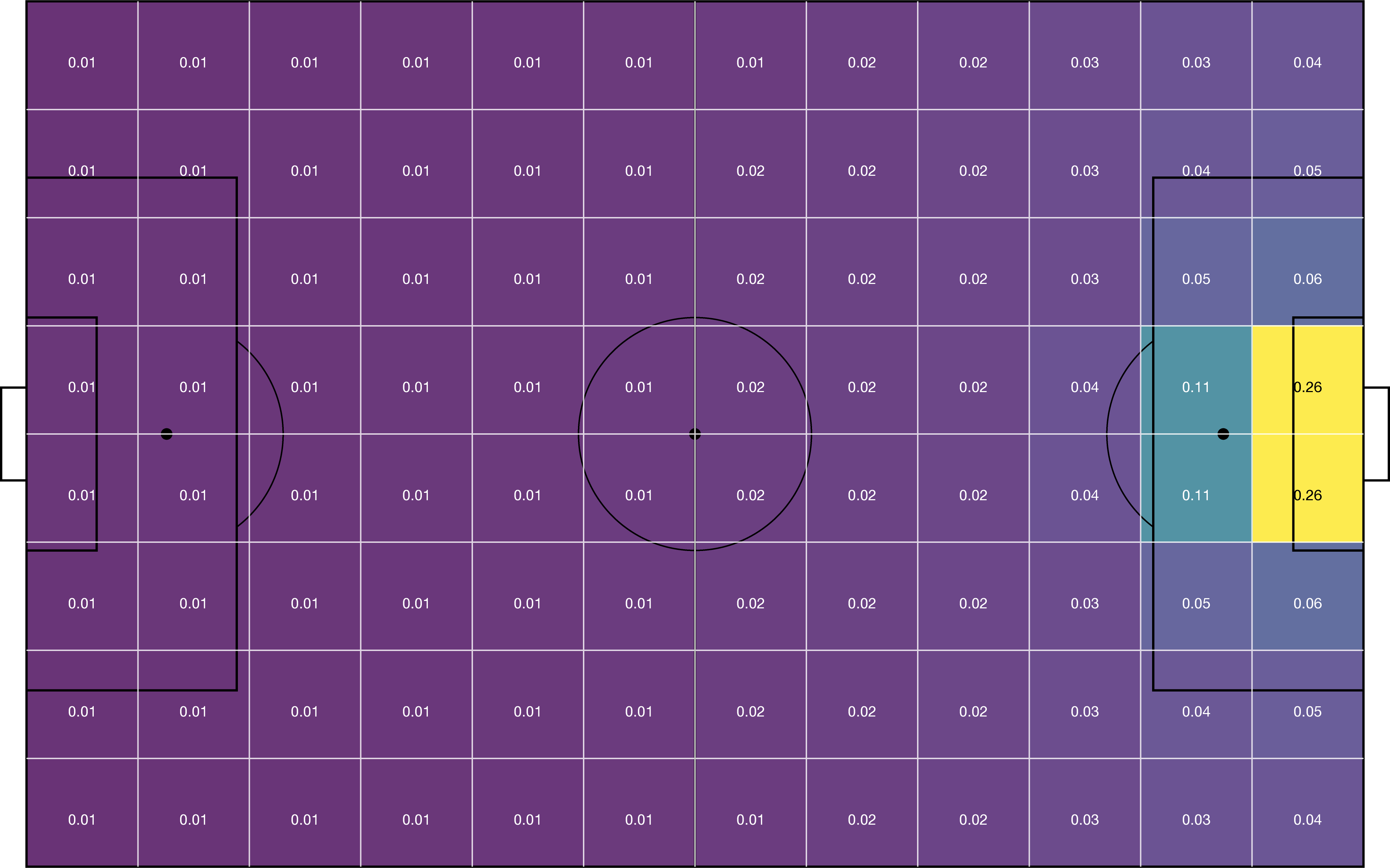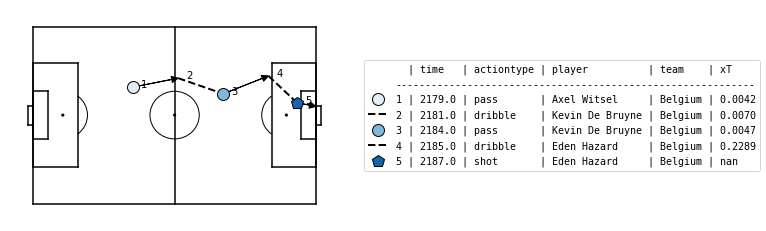# Quickstart#

Eager to get started valuing some soccer actions? This page gives a quick introduction in how to get started.

## Installation#

First, make sure that socceraction is installed:

\$ pip install socceraction


For detailed instructions and other installation options, check out our detailed installation instructions.

## Data#

First of all, you will need some data. Luckily, both StatsBomb and Wyscout provide a small freely available dataset. The data module of socceraction makes it trivial to load these datasets as Pandas dataframes. In this short introduction, we will work with Statsbomb’s dataset of the 2018 World Cup.

import pandas as pd

# Set up the StatsBomb data loader

# View all available competitions
df_competitions = SBL.competitions()

# Create a dataframe with all games from the 2018 World Cup
df_games = SBL.games(competition_id=43, season_id=3).set_index("game_id")


Note

Keep in mind that by using the public StatsBomb data you are agreeing to their user agreement.

For each game, you can then retrieve a dataframe containing the teams, all players that participated, and all events that were recorded in that game. Specifically, we’ll load the data from the third place play-off game between England and Belgium.

game_id = 8657
df_teams = SBL.teams(game_id)
df_players = SBL.players(game_id)
df_events = SBL.events(game_id)


The event stream format is not well-suited for data analysis: some of the recorded information is irrelavant for valuing actions, each vendor uses their own custom format and definitions, and the events are stored as unstructured JSON objects. Therefore, socceraction uses the SPADL format for describing actions on the pitch. With the code below, you can convert the events to SPADL actions.

import socceraction.spadl as spadl

home_team_id = df_games.at[game_id, "home_team_id"]


With the matplotsoccer package, you can try plotting some of these actions:

import matplotsoccer as mps

# Select relevant actions
df_actions_goal = df_actions.loc[2197:2201]
# Replace result, actiontype and bodypart IDs by their corresponding name
# Add team and player names
df_actions_goal = df_actions_goal.merge(df_teams).merge(df_players)
# Create the plot
mps.actions(
location=df_actions_goal[["start_x", "start_y", "end_x", "end_y"]],
action_type=df_actions_goal.type_name,
team=df_actions_goal.team_name,
result=df_actions_goal.result_name == "success",
label=df_actions_goal[["time_seconds", "type_name", "player_name", "team_name"]],
labeltitle=["time", "actiontype", "player", "team"],
zoom=False
)


## Valuing actions#

We can now assign a numeric value to each of these individual actions that quantifies how much the action contributed towards winning the game. Socceraction implements two frameworks for doing this: xT and VAEP.

### Valuing actions with xT#

The expected threat or xT model overlays a $$M \times N$$ grid on the pitch in order to divide it into zones. Each zone $$z$$ is then assigned a value $$xT(z)$$ that reflects how threatening teams are at that location, in terms of scoring. An example grid is visualized below.The code below allows you to load league-wide xT values from the 2017-18 Premier League season (the 12x8 grid shown above). Instructions on how to train your own model can be found in the detailed documentation about xT.

import socceraction.xthreat as xthreat

url_grid = "https://karun.in/blog/data/open_xt_12x8_v1.json"


Subsequently, the model can be used to value actions that successfully move the ball between two zones by computing the difference between the threat value on the start and end location of each action. The xT framework does not assign a value to failed actions, shots and defensive actions such as tackles.

df_actions_ltr = spadl.play_left_to_right(df_actions, home_team_id)
df_actions["xT_value"] = xT_model.rate(df_actions_ltr)### Valuing actions with VAEP#

VAEP goes beyond the possession-based approach of xT by trying to value a broader set of actions and by taking the action and game context into account. Therefore, VAEP frames the problem of quantifying a soccer player’s contributions within a game as a binary classification task and rates each action by estimating its effect on the short-term probabilities that a team will both score or concede. That is, VAEP quanitifies the effect of an action $$a_i$$ that moves the game from state $$S_{i−1} = \{a_{i-n}, \ldots, a_{i−1}\}$$ to state $$S_i = \{a_{i-n+1}, . . . , a_{i−1}, a_i\}$$, where each game state is represented by the $$n$$ previous actions. Then each game state is represented using a set of features and assigned two labels. A first label that defines whether the team in possession scored a goal in the next $$k$$ actions; a second label that defines whether the team in possession conceded a goal in the next $$k$$ actions.

This allows to train two classifiers: one that predicts the probability that a team will score in the next $$k$$ actions from the current game state ($$P_{scores}$$) and one that predicts the probability that a team will concede in the next $$k$$ actions from the current game state ($$P_{concedes}$$).

from socceraction.vaep import VAEP
from tqdm import tqdm

VAEP_model = VAEP(nb_prev_actions=1)

# compute features and labels for each game
all_features, all_labels = [], []
for game_id, game in tqdm(list(df_games.iterrows())):
game_events = SBL.events(game_id)
# convert the events to actions
game_home_team_id = df_games.at[game_id, "home_team_id"]
# compute features and labels
all_features.append(VAEP_model.compute_features(game, game_actions))
all_labels.append(VAEP_model.compute_labels(game, game_actions))
# combine all features and labels in a single dataframe
all_features = pd.concat(all_features)
all_labels = pd.concat(all_labels)

# fit the model
VAEP_model.fit(all_features, all_labels)


Given these probabilites, VAEP estimates the risk-reward trade-off of an action as the sum of the offensive value $$\Delta P_\textrm{score}(a_{i})$$ (i.e., how much did the action increase the probability of scoring) and defensive value $$- \Delta P_\textrm{concede}(a_{i})$$ (i.e., how much did the action decrease the probability of conceding) of the action: $$\textrm{VAEP}(a_i) = \Delta P_\textrm{score}(a_{i}) - \Delta P_\textrm{concede}(a_{i})$$.

# rate a game
ratings = VAEP_model.rate(games.loc[game_id], actions)Ready for more? Check out the detailed documentation about the data representation and action value frameworks.